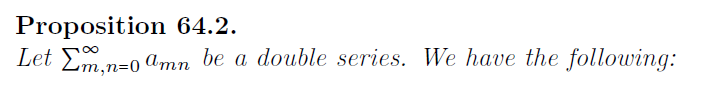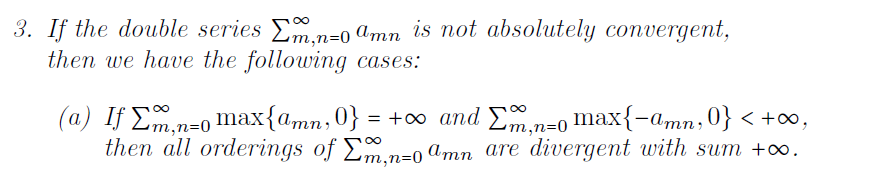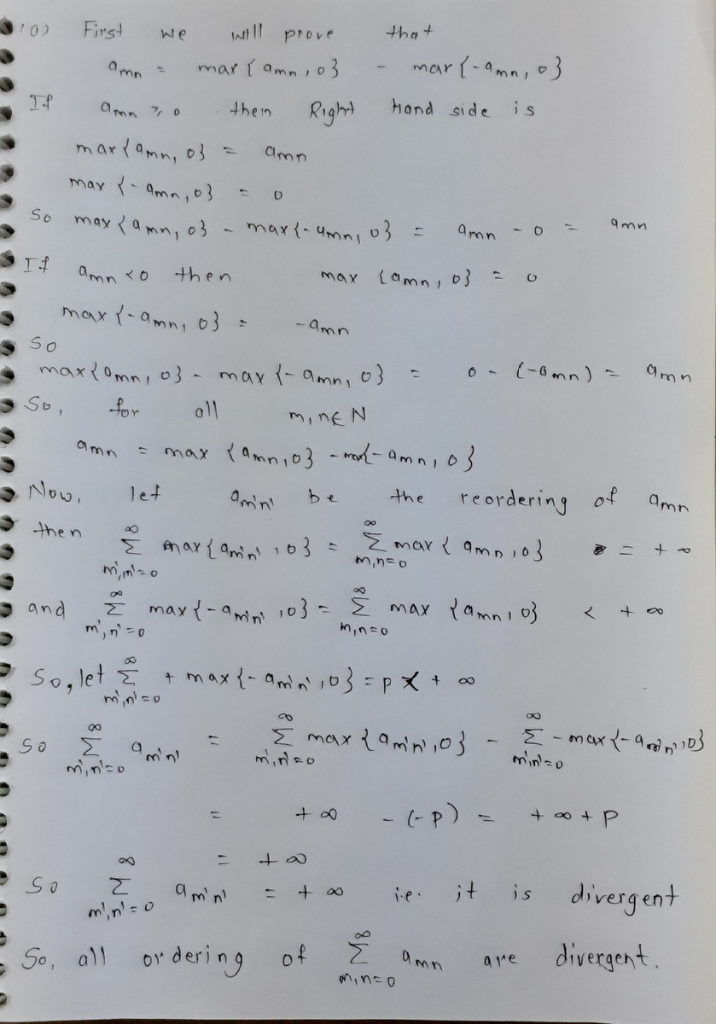# Prove Proposition 64.2 m.n-0 amn be a double series. We have the following: 3. If the double series Σ n n-00mn is not...Prove

Proposition 64.2 m.n-0 amn be a double series. We have the following:
3. If the double series Σ n n-00mn is not absolutely convergent. then we have the following cases: If Σοο , n-0 max(@mn. 0): +oo and Σοο , n-0 max(-amn. 0} (a) +oo. m,n##### Add Answer of: Prove Proposition 64.2 m.n-0 amn be a double series. We have the following: 3. If the double series Σ n n-00mn is not...
More Homework Help Questions Additional questions in this topic.